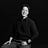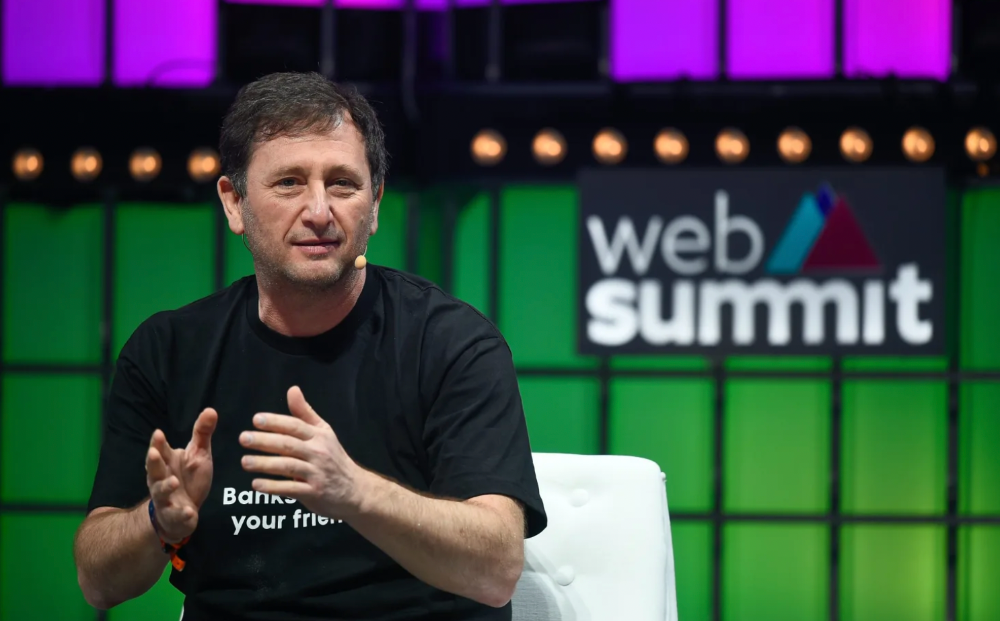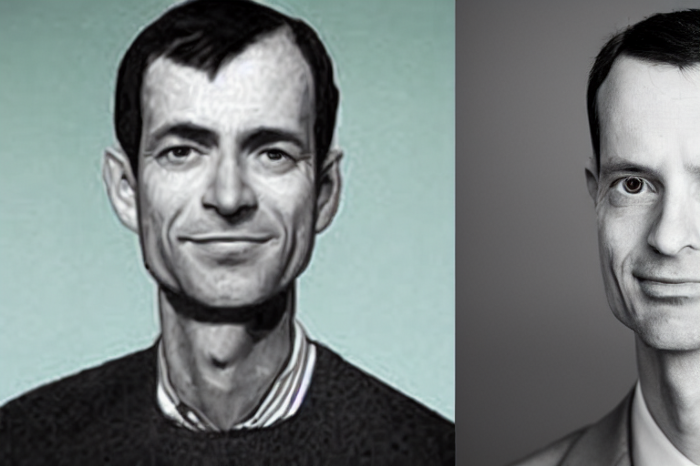Ben

7 months ago

## More on Web3 & CryptoVitalik

1 year ago

## An approximate introduction to how zk-SNARKs are possible (part 2)

If tasked with the problem of coming up with a zk-SNARK protocol, many people would make their way to this point and then get stuck and give up. How can a verifier possibly check every single piece of the computation, without looking at each piece of the computation individually? But it turns out that there is a clever solution.

## Polynomials

Polynomials are a special class of algebraic expressions of the form:

• x+5
• x^4
• x^3+3x^2+3x+1
• 628x^{271}+318x^{270}+530x^{269}+…+69x+381

i.e. they are a sum of any (finite!) number of terms of the form cx^k

There are many things that are fascinating about polynomials. But here we are going to zoom in on a particular one: polynomials are a single mathematical object that can contain an unbounded amount of information (think of them as a list of integers and this is obvious). The fourth example above contained 816 digits of tau, and one can easily imagine a polynomial that contains far more.

Furthermore, a single equation between polynomials can represent an unbounded number of equations between numbers. For example, consider the equation A(x)+ B(x) = C(x). If this equation is true, then it's also true that:

• A(0)+B(0)=C(0)
• A(1)+B(1)=C(1)
• A(2)+B(2)=C(2)
• A(3)+B(3)=C(3)

And so on for every possible coordinate. You can even construct polynomials to deliberately represent sets of numbers so you can check many equations all at once. For example, suppose that you wanted to check:

• 12+1=13
• 10+8=18
• 15+8=23
• 15+13=28

You can use a procedure called Lagrange interpolation to construct polynomials A(x) that give (12,10,15,15) as outputs at some specific set of coordinates (eg. (0,1,2,3)), B(x) the outputs (1,8,8,13) on thos same coordinates, and so forth. In fact, here are the polynomials:

• A(x)=-2x^3+\frac{19}{2}x^2-\frac{19}{2}x+12
• B(x)=2x^3-\frac{19}{2}x^2+\frac{29}{2}x+1
• C(x)=5x+13

Checking the equation A(x)+B(x)=C(x) with these polynomials checks all four above equations at the same time.

## Comparing a polynomial to itself

You can even check relationships between a large number of adjacent evaluations of the same polynomial using a simple polynomial equation. This is slightly more advanced. Suppose that you want to check that, for a given polynomial F, F(x+2)=F(x)+F(x+1) with the integer range {0,1…89} (so if you also check F(0)=F(1)=1, then F(100) would be the 100th Fibonacci number)

As polynomials, F(x+2)-F(x+1)-F(x) would not be exactly zero, as it could give arbitrary answers outside the range x={0,1…98}. But we can do something clever. In general, there is a rule that if a polynomial P is zero across some set S=\{x_1,x_2…x_n\} then it can be expressed as P(x)=Z(x)*H(x), where Z(x)=(x-x_1)*(x-x_2)*…*(x-x_n) and H(x) is also a polynomial. In other words, any polynomial that equals zero across some set is a (polynomial) multiple of the simplest (lowest-degree) polynomial that equals zero across that same set.

Why is this the case? It is a nice corollary of polynomial long division: the factor theorem. We know that, when dividing P(x) by Z(x), we will get a quotient Q(x) and a remainder R(x) is strictly less than that of Z(x). Since we know that P is zero on all of S, it means that R has to be zero on all of S as well. So we can simply compute R(x) via polynomial interpolation, since it's a polynomial of degree at most n-1 and we know n values (the zeros at S). Interpolating a polynomial with all zeroes gives the zero polynomial, thus R(x)=0 and H(x)=Q(x).

Going back to our example, if we have a polynomial F that encodes Fibonacci numbers (so F(x+2)=F(x)+F(x+1) across x=\{0,1…98\}), then I can convince you that F actually satisfies this condition by proving that the polynomial P(x)=F(x+2)-F(x+1)-F(x) is zero over that range, by giving you the quotient:
H(x)=\frac{F(x+2)-F(x+1)-F(x)}{Z(x)}
Where Z(x) = (x-0)*(x-1)*…*(x-98).
You can calculate Z(x) yourself (ideally you would have it precomputed), check the equation, and if the check passes then F(x) satisfies the condition!

Now, step back and notice what we did here. We converted a 100-step-long computation into a single equation with polynomials. Of course, proving the N'th Fibonacci number is not an especially useful task, especially since Fibonacci numbers have a closed form. But you can use exactly the same basic technique, just with some extra polynomials and some more complicated equations, to encode arbitrary computations with an arbitrarily large number of steps.

see part 3

Olga Kharif

10 months ago

## A month after freezing customer withdrawals, Celsius files for bankruptcy.Alex Mashinsky, CEO of Celsius, speaks at Web Summit 2021 in Lisbon.

Celsius Network filed for Chapter 11 bankruptcy a month after freezing customer withdrawals, joining other crypto casualties.

Celsius took the step to stabilize its business and restructure for all stakeholders. The filing was done in the Southern District of New York.

The smallest was Ping. Ping's compensation was the smallest. Andre Duand owned 2% but was worth $20m at IPO. That's less than some billion-dollar paydays, but still good. IPOs can be lucrative, as you can see. Preserving equity could be the difference between a$20mm and $15bln payday (Coinbase).The woman 6 months ago ## The renowned and highest-paid Google software engineer His story will inspire you.“Google search went down for a few hours in 2002; Jeff Dean handled all the queries by hand and checked quality doubled.”- Jeff Dean Facts. One of many Jeff Dean jokes, but you get the idea. Google's top six engineers met in a war room in mid-2000. Google's crawling system, which indexed the Web, stopped working. Users could still enter queries, but results were five months old. Google just signed a deal with Yahoo to power a ten-times-larger search engine. Tension rose. It was crucial. If they failed, the Yahoo agreement would likely fall through, risking bankruptcy for the firm. Their efforts could be lost. A rangy, tall, energetic thirty-one-year-old man named Jeff dean was among those six brilliant engineers in the makeshift room. He had just left D. E. C. a couple of months ago and started his career in a relatively new firm Google, which was about to change the world. He rolled his chair over his colleague Sanjay and sat right next to him, cajoling his code like a movie director. The history started from there. When you think of people who shaped the World Wide Web, you probably picture founders and CEOs like Larry Page and Sergey Brin, Marc Andreesen, Tim Berners-Lee, Bill Gates, and Mark Zuckerberg. They’re undoubtedly the brightest people on earth. Under these giants, legions of anonymous coders work at keyboards to create the systems and products we use. These computer workers are irreplaceable. ## Let's get to know him better. It's possible you've never heard of Jeff Dean. He's American. Dean created many behind-the-scenes Google products. Jeff, co-founder and head of Google's deep learning research engineering team, is a popular technology, innovation, and AI keynote speaker. While earning an MS and Ph.D. in computer science at the University of Washington, he was a teaching assistant, instructor, and research assistant. Dean joined the Compaq Computer Corporation Western Research Laboratory research team after graduating. Jeff co-created ProfileMe and the Continuous Profiling Infrastructure for Digital at Compaq. He co-designed and implemented Swift, one of the fastest Java implementations. He was a senior technical staff member at mySimon Inc., retrieving and caching electronic commerce content. Dean, a top young computer scientist, joined Google in mid-1999. He was always trying to maximize a computer's potential as a child. ## An expert His high school program for processing massive epidemiological data was 26 times faster than professionals'. Epi Info, in 13 languages, is used by the CDC. He worked on compilers as a computer science Ph.D. These apps make source code computer-readable. Dean never wanted to work on compilers forever. He left Academia for Google, which had less than 20 employees. Dean helped found Google News and AdSense, which transformed the internet economy. He then addressed Google's biggest issue, scaling. Growing Google faced a huge computing challenge. They developed PageRank in the late 1990s to return the most relevant search results. Google's popularity slowed machine deployment. Dean solved problems, his specialty. He and fellow great programmer Sanjay Ghemawat created the Google File System, which distributed large data over thousands of cheap machines. These two also created MapReduce, which let programmers handle massive data quantities on parallel machines. They could also add calculations to the search algorithm. A 2004 research article explained MapReduce, which became an industry sensation. ## Several revolutionary inventions Dean's other initiatives were also game-changers. BigTable, a petabyte-capable distributed data storage system, was based on Google File. The first global database, Spanner, stores data on millions of servers in dozens of data centers worldwide. It underpins Gmail and AdWords. Google Translate co-founder Jeff Dean is surprising. He contributes heavily to Google News. Dean is Senior Fellow of Google Research and Health and leads Google AI. ## Recognitions The National Academy of Engineering elected Dean in 2009. He received the 2009 Association for Computing Machinery fellowship and the 2016 American Academy of Arts and Science fellowship. He received the 2007 ACM-SIGOPS Mark Weiser Award and the 2012 ACM-Infosys Foundation Award. Lists could continue. A sneaky question may arrive in your mind: How much does this big brain earn? Well, most believe he is one of the highest-paid employees at Google. According to a survey, he is paid$3 million a year.

He makes espresso and chats with a small group of Googlers most mornings. Dean steams milk, another grinds, and another brews espresso. They discuss families and technology while making coffee. He thinks this little collaboration and idea-sharing keeps Google going.

“Some of us have been working together for more than 15 years,” Dean said. “We estimate that we’ve collectively made more than 20,000 cappuccinos together.”

We all know great developers and software engineers. It may inspire many.